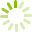# Fundamentals of Mathematics 5 (MATH 0651)

MATH 0651 introduces students to skills, concepts, vocabulary, and problem solving strategies for working with common fractions. Emphasis is placed on understanding proper and improper fractions, mixed numbers, and equivalent fractions; adding, subtracting, multiplying and dividing fractions; and comparing common fractions and decimal fractions. This course is the fifth of six levels of Basic Education Mathematics and will prepare students to meet personal, career, or further academic goals.
Course code: MATH 0651
Credits: 0.0
Length: 156.0 hours
Course outline: view https://www.vcc.ca/vccphp/courseoutline?subject=MATH&number=0651

## Prerequisites

MATH 0300.